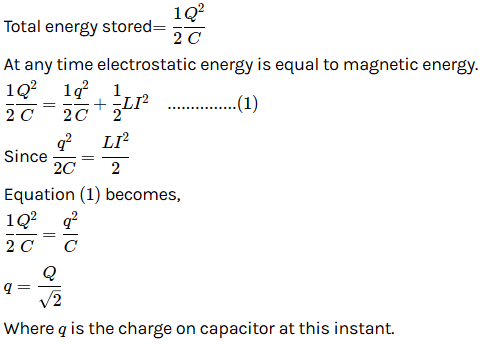# In an oscillating LC circuit the maximum charge on the capacitor is Q . The charge on the capacitor when the energy is stored equally between the electric and magnetic field is(a) Q/2(b) Q/ v3(c) Q / v2(d) QNavjyot Kalra
8 years ago

(c) When the capacitor is completely charged, the total energy in the L.C circuit is with the capacitor and that energy is E =1/2 Q2/C

When half energy is with the capacitor in the form of electric field between the plates of the capacitor we get E/2 = ½ Q2/C where Q’ is the charge on one plate of the capacitor

? ½ x ½ Q2 /C = ½ Q2/C ? Q’ = Q / v2

4 years ago
It is saying to find charge when both the fields become equal.
so initially capacitor possess full PE .
It is being converted to magnetic energy.
Hence when capacitor energy becomes half then only both the energies become equal.
So capacitor energy becomes half and we know that PE is directly proportional to square of Q so it will become root 2 times.Kushagra Madhukar